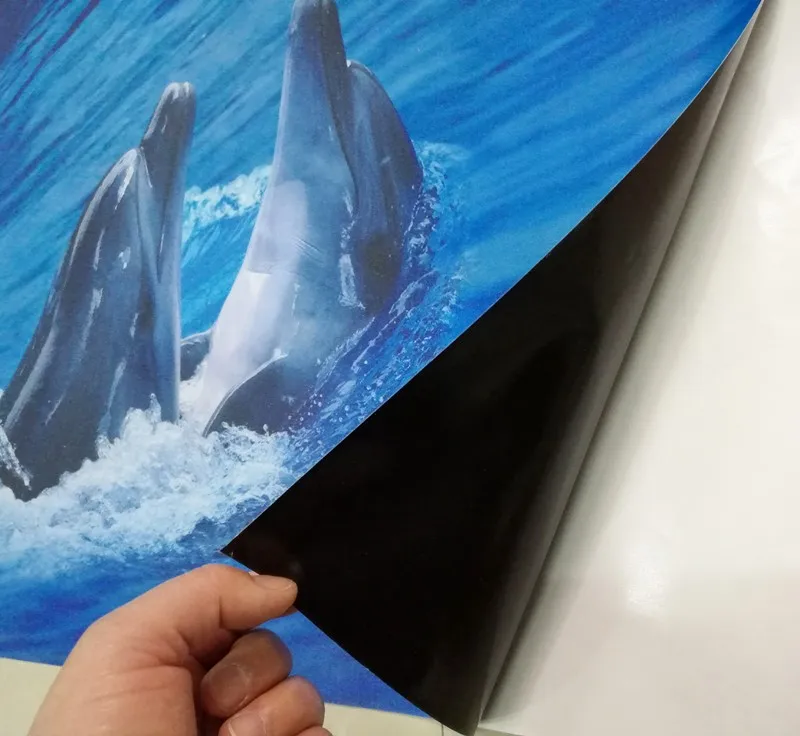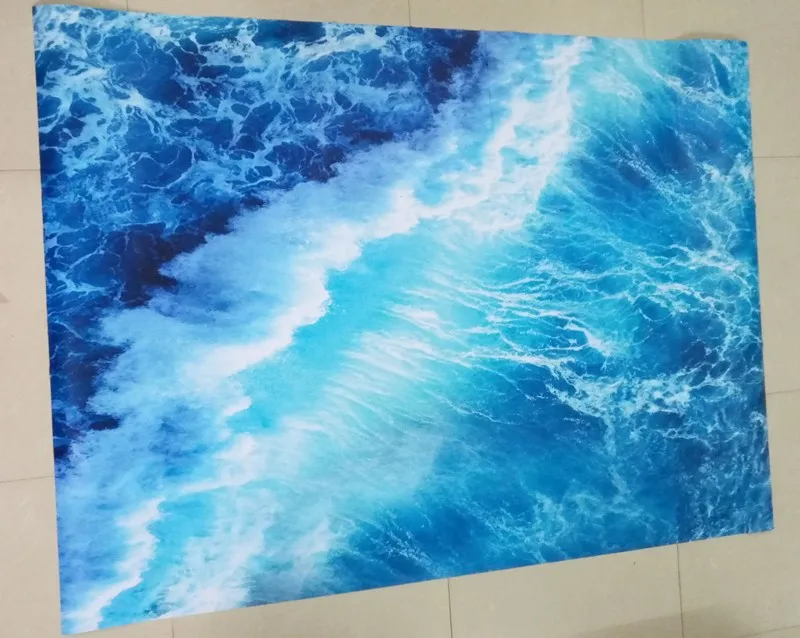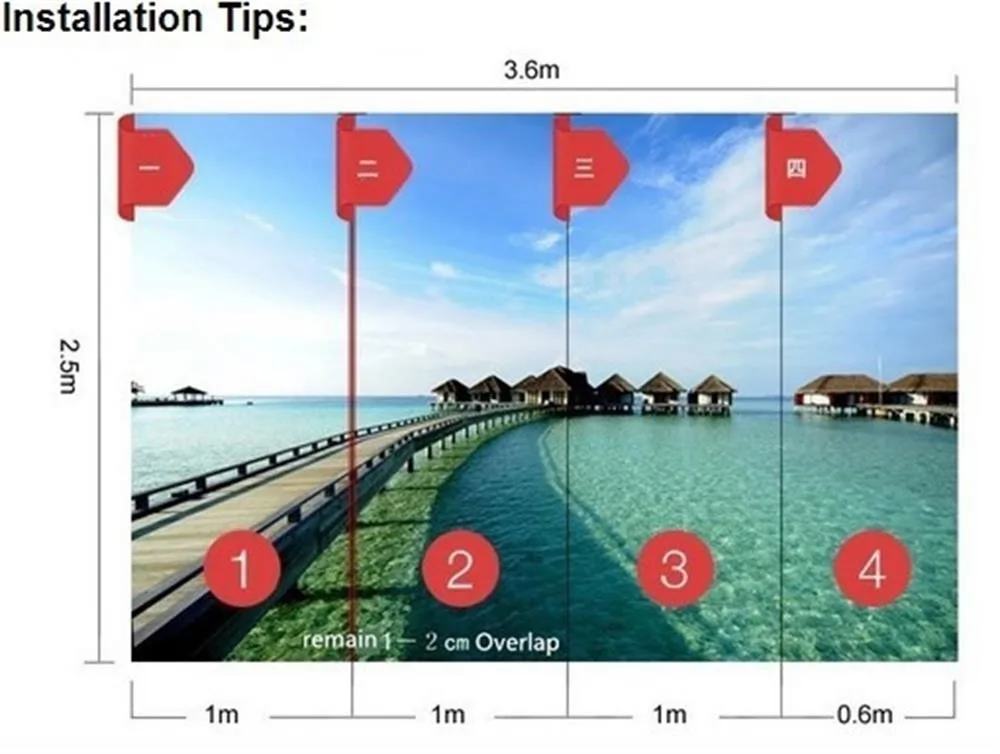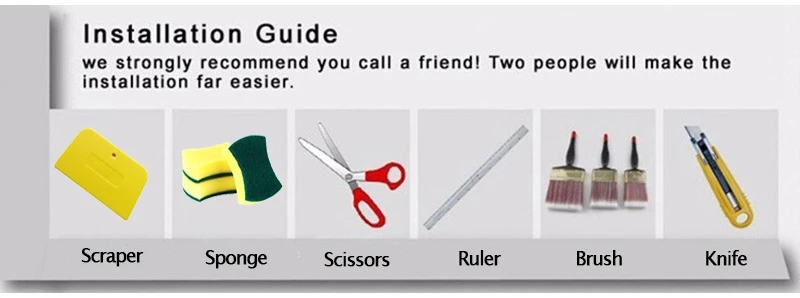# 3D Waterfalls Landscape Floor Tiles PVC Waterproof  (1 ㎡)  Mural Wall Floor Stickers

Save \$29.00

Price:
Sale price\$39.99 Regular price\$68.99

# 3D Waterfalls Landscape Floor Tiles PVC Waterproof (1 ㎡)  Mural Wall Floor Stickers

## If we haven't got your exact size after 24 hours,We will arrange them for producing according to the default size.

If you need 6 square meters, please choose "1 square meter" and "Quantity 6" here.

Default size :

Quantity 1 :     1 square meter=140cm(L) x 70cm(W) (4'7" x 2'4") ft

Quantity 2:      2 square meter=200cm(L) x 100cm(W) (6'7" x 3'3") ft

Quantity 3:      3 square meter=208cm(L) x 146cm(W) (6'9" x 4'9") ft

Quantity 4:      4 square meter=200cm(L) x 200cm(W) (6'7" x 6'7") ft

Quantity 5:      5 square meter=250cm(L) x 200cm(W) (8'2" x 6'7") ft

Quantity 6:      6 square meter=300cm(L) x 200cm(W) (9'10" x 6'7") ft

Quantity 7:      7 square meter=300cm(L) x 250cm(W) (9'10" x 8'2") ft

Quantity 8:      8 square meter=400cm(L) x 200cm(W) (13'1" x 6'7") ft

Quantity 9:      9 square meter=350cm(L) x 260cm(W) (11'5" x 8'6") ft

Quantity 10:   10 square meter=360cm(L) x 280cm(W) (11'10" x 9'2") ft

Quantity 12:   12 square meter=400cm(L) x 300cm(W) (13'1" x 9'10") ft

Quantity 16:   16 square meter=500cm(L) x 320cm(W) (16'5" x 10'6") ft

Product show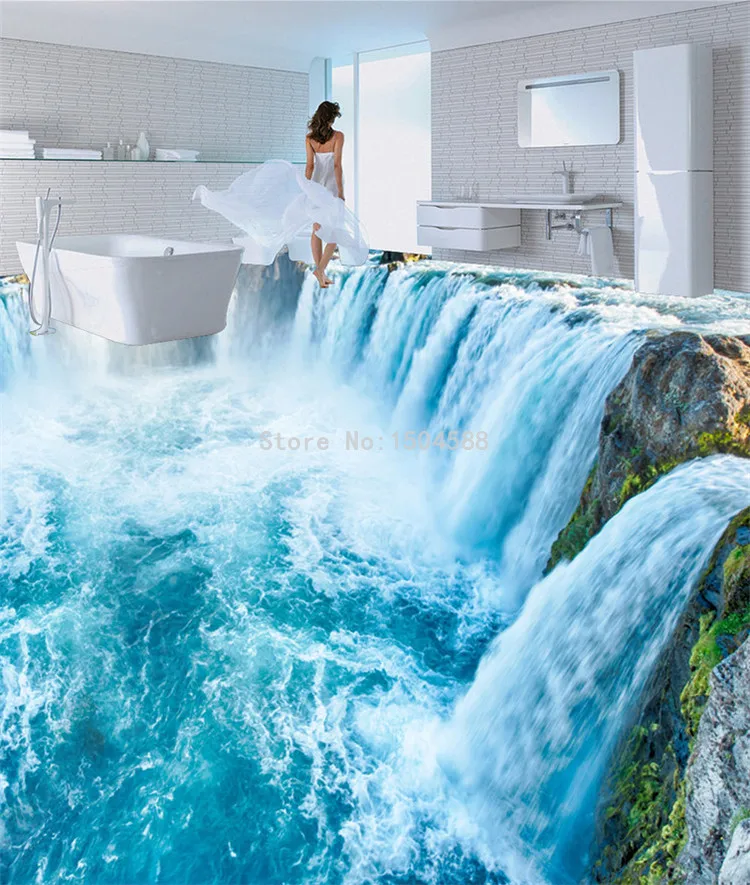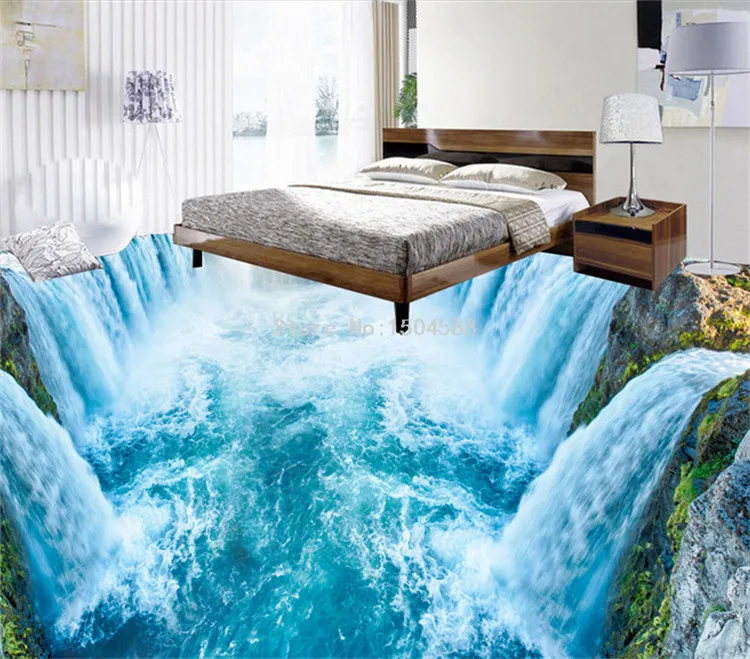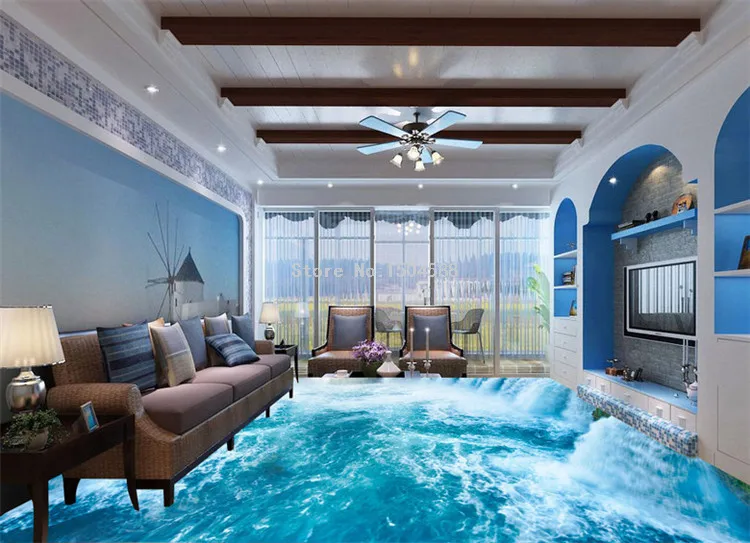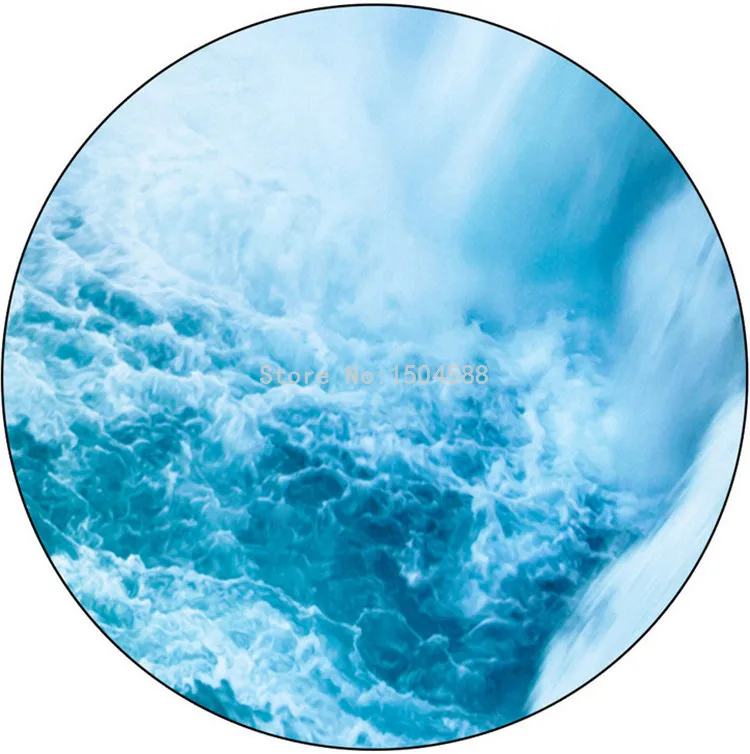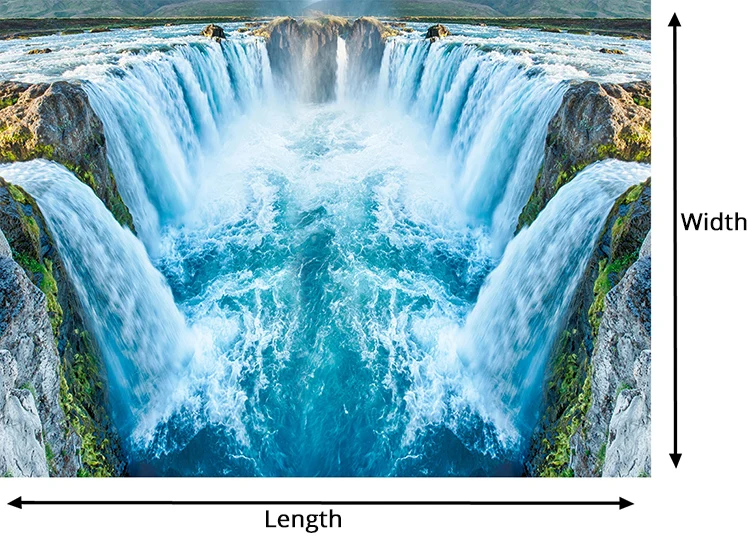Super thick. Waterproof.  Skidproof  PVC Self-adhesive Floor Sticker